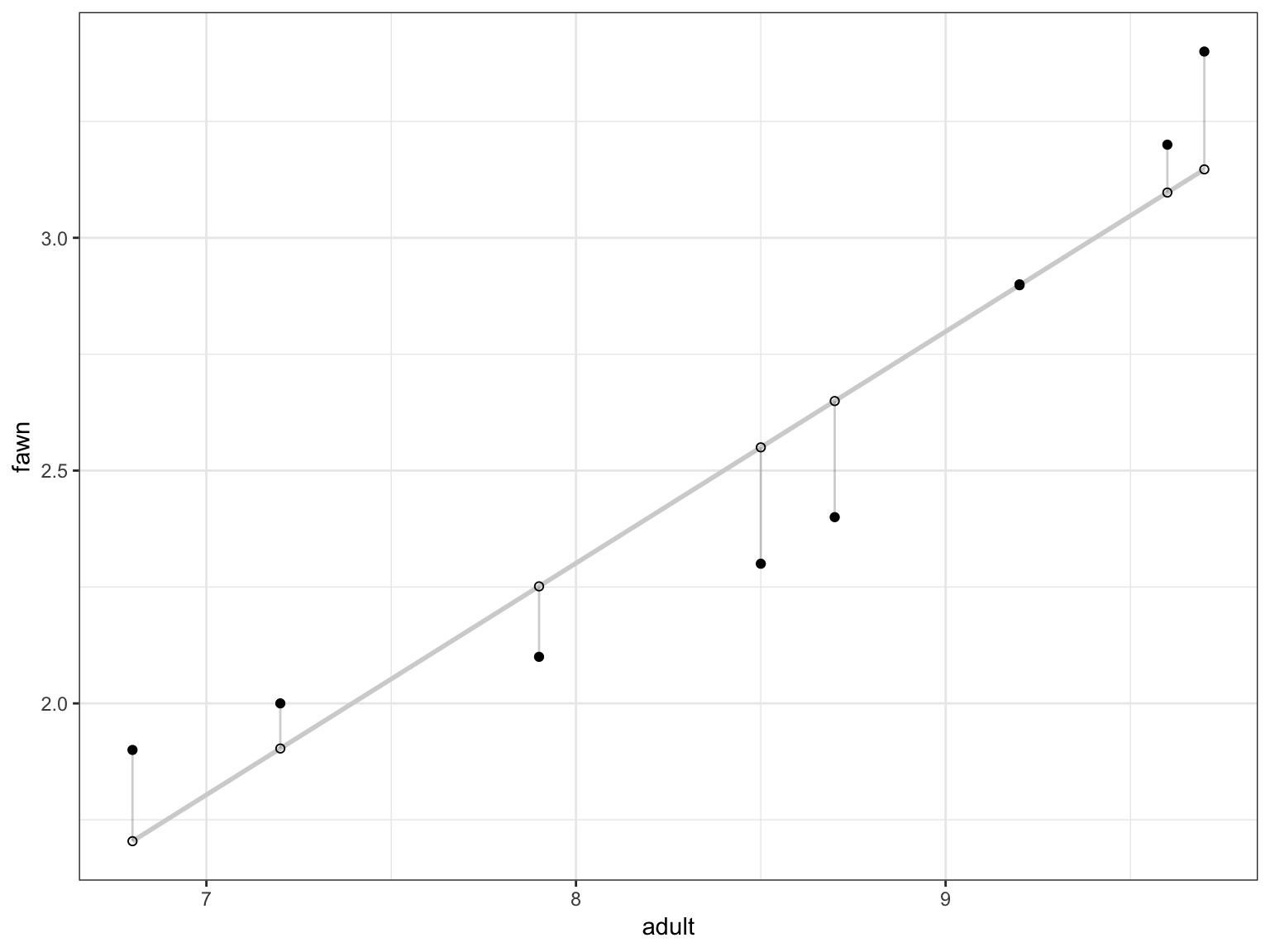# IST 687: Linear Modeling

2019-08-28 17:37:01

## Linear Regression

• An algorithm for prediction or explaination “…is variable X associated with variable Y? If so, what the relationship and can we use it to predict Y

Y = dependent variable/ outcome/ target
X = independent variable/ attribute/ feature

• Sample problems: consumer spending and GDP, hours studying and test scores, fawn population and adults (homework)

## Simple Linear Regression

• In R… if we wanted to find the “best fit line” to predict the outcome variable fawn as a function of the predictor variable adult

• The fawn dataset contains information about the number of fawns born over eight spring seasons and includes the number of adult Antelopes, precipitation, and the severity of winter

##   fawn adult precipitation severity
## 1  2.9   9.2          13.2        2
## 2  2.4   8.7          11.5        3
## 3  2.0   7.2          10.8        4
## 4  2.3   8.5          12.3        2
## 5  3.2   9.6          12.6        3
## 6  1.9   6.8          10.6        5

## Simple Linear Regression

• Our goal is predict the number of fawn given the number of adults so we can forecast Antelope population. The intuition is that the number of adults is a good indicator of the number of fawns to be born.

## Simple Linear Regression (vs. correlation)

• Determines the association of two variables, but no indication of their numerical depedency

cor.test(populations$adult,populations$fawn)

##
##  Pearson's product-moment correlation
##
## data:  populations$adult and populations$fawn
## t = 6.6757, df = 6, p-value = 0.0005471
## alternative hypothesis: true correlation is not equal to 0
## 95 percent confidence interval:
##  0.6917446 0.9891217
## sample estimates:
##       cor
## 0.9387973

## The “best fit” line## The linear model

“Predict the fawn population based on the numebr of adults”

model <- lm(formula = fawn ~ adult, data=fawns) summary(model)

FYI: A multiple linear regression might account for other factors in the data lm(formula = fawn ~ adult + precipitation, data=fawns)

## Fitting the Model

##
## Call:
## lm(formula = fawn ~ adult, data = populations)
##
## Residuals:
##      Min       1Q   Median       3Q      Max
## -0.24988 -0.17586  0.04938  0.12611  0.25309
##
## Coefficients:
##             Estimate Std. Error t value Pr(>|t|)
## (Intercept) -1.67914    0.63422  -2.648 0.038152 *
## adult        0.49753    0.07453   6.676 0.000547 ***
## ---
## Signif. codes:  0 '***' 0.001 '**' 0.01 '*' 0.05 '.' 0.1 ' ' 1
##
## Residual standard error: 0.2121 on 6 degrees of freedom
## Multiple R-squared:  0.8813, Adjusted R-squared:  0.8616
## F-statistic: 44.56 on 1 and 6 DF,  p-value: 0.0005471

## What the model predicted and errors

##   fawn adult predicted    residuals
## 1  2.9   9.2  2.898148  0.001851932
## 2  2.4   8.7  2.649383 -0.249382596
## 3  2.0   7.2  1.903086  0.096913724
## 4  2.3   8.5  2.549877 -0.249876646
## 5  3.2   9.6  3.097161  0.102839413
## 6  1.9   6.8  1.704074  0.195925887

## Interpreting the Output - Coefficients

Coefficients: Represent the intercept and slope terms in the linear model.

Intercept: The expected value of y when we all other variables are held constant (Predict number of fawns if there were 0 adults is -1.6791364)

Slope: The effect the independent variable has on the outcome.(For each one unit increase in the number of adult the number of fawns increases (or decreases if the estimate is negative) by 0.4975309)

## (Intercept)       adult
##  -1.6791364   0.4975309

## Interpreting the Output - Coefficients

$$\mathbf{p-value}$$: indicates the extent to which a coefficient is statistically significant.

• Interpret $$p-value$$ as the probability that, given a chance model, results as extreme as the observed results could occur

• Lower p-values are better and the cutoff for significance is normally $$<=$$ 0.05, but may vary depending on field of study.

## Interpreting the Output - Model Performance

Two approaches to assess the overlall model:

$$\mathbf{p-value}$$: indicates the extent to which a coefficient is statistically significant. - We can consider a linear model to be statistically significant only when both these p-Values are less that the pre-determined statistical significance level

$$\mathbf{R^2}$$ (coefficient of determination): ranges from 0 to 1 and measures the proportion of variation in data accounted for in the model. “How well the model fits the data”.

• In fawn model $$R^2$$ = 0.8813404 and adjusted $$R^2$$ = 0.8615638

## Reporting/interpreting the results of simple linear regression

• An example of explaining the relationship between fawn and adults to be written in text:

“In modeling the fawns population it was found that the number adults ($$\beta$$ = 0.5, p < .001) was a significant predictor. The overall model fit was $$R^2$$ = 0.88.”

• Reporting/communicating results examples: A few templates

• An example of forecasting future fawn populations

• Predicting number of fawns with 5 adults $\hat{y} = -1.68 + 0.5*5$ $0.82 = -1.68+2.5$

## Other important points

• Assumptions associated with linear modeling: Comprehensive list
• Normality assumption (use of Q-Q plots)
• Dealing with outliers (alternatives: weighed regression, removing outliers, etc.)
• Multi-collinearity
• Interpreting coefficients with factors e.g., male/female as indepenent variables